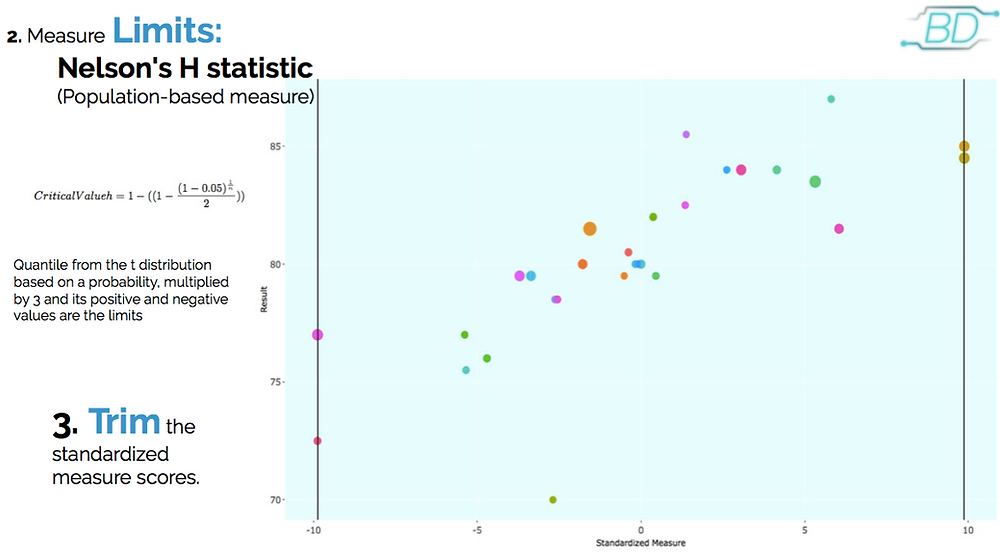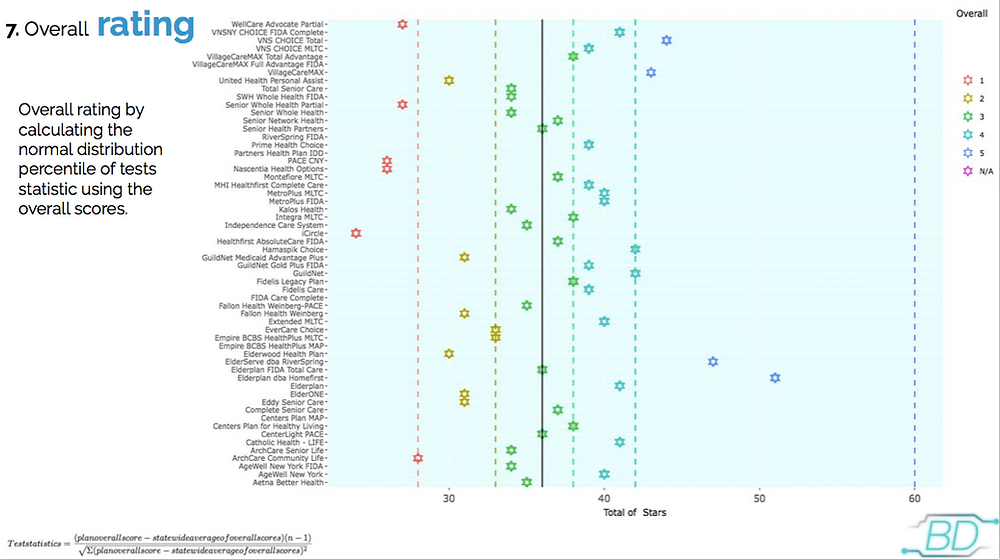Search

# MLTC & Healthcare: Star-Rating for MLTC plans

Updated: Jun 30, 2020What is The Managed Long-Term Care (MLTC) performance data?

It is a semi-annual evaluation of New York state- certified MLTC plans. Rates are calculated for each performance measure by the plan, and describe their population or assess their quality of care.

Plans are evaluated on the quality of care they provide and on enrollees’ satisfaction .

Type of performance data.

Different type of measures, survey-based measures or population-based measures across all plans in MLTC.

The results are reported per semester (in this case 2018), and there is a process where, depending on the results of these measures, a final grade is assigned to each plan (stars).

Areas, Domains and Measures.

There are a total of 18 measures, grouped in 12 domains, distributed in 4 areas. Here we present a sample of measures grouped by Domains and Areas. We can notice that different domains can contain a single measure or more.New York State Department of Health Managed Long-Term Care (MLTC) Consumer Guide 2018.

The process for Star rating per plan

The star-rating process consists of 7 steps. The first 3 steps are related to the measures, this means we only do some calculation on the result of the single measures.

The last 4 steps are carried out at the domain level. This means that if a domain only has one measure we keep the result of the first 3 steps. On the other hand, if the domain contains 2 or more measures after step number 3, we will average over the total number of measures in the domain.Example

We will work with the area Stability or Improvement, this area contains 4 domains: ADL Stable or Improved, Pain Intensity Stable or Improved, Shortness of Breath Stable or Improved and Urinary Continence Stable or Improved.

In this specific case, all the domains have only one measure. For simplicity, we will use the domain Urinary Continence Stable or Improved to exemplify the calculation process.Standardization of measures

Here it is important the distinction between the two types of data. When the measure we are working with is population-based measure, we need to use Nelson's H-statistics to make the calculations. When the measure is a survey-based measure we use Student's t-statistic.

In the calculation, the difference resides, among other characteristics, on the variables we use to calculate steps 1 and 2. In both cases, we will use plan rate and statewide rates as variables. Nevertheless, when the measure is survey-based measure, the standardization is carried out by subtracting from the plan rate the statewide rate and that result goes over the standard error.

When the measure is population-based measure, the formula is a little more complex, and it involves the denominator of the measure. The denominator is the total of members that qualify for a specific measure. Here the size of the plan can contribute to improving the rating or lowering it.From the table on the left hand side, planrate is represented by the column Result and plandenominator by the column Denominator. From the right hand side table we can obtain the statewide rate and the statewide denominator. It is easy to see that the statewide denominator is (must be) always greater than any plan denominator.Here we can see the transformation, from the original results to the standard measure. The size of the dots are related to the denominator of each plan, i.e if the size is relatively small, this plan has a small denominator.

Measure limits and trimming

Once again, in this part of the calculation, since we are working with a population-based measure to find the limits of the trimming process is carried out using Nelson's H-statistics.

In this case, the limits are the quantile from the t distribution based on a probability, multiplied by 3 and its positive and negative values are the limits. The probability is obtained using the formula on the left-hand side, where n is the total of plans participating in this specific measure.The solid black lines represent the limits (upper and lower). These limits are established in order to make the distribution more compact. For example, the dots that appeared on top of the lines mean that those plans got a higher standard measure score, and they were pulled back to that limit (right-hand side solid black line). On the left-hand side, it means that those plans on top of the line obtained the lowest standard measures scores and they were brought up to the lower limit.

Until now, we have been working with the results of every measure. And now it is the time to calculate the total stars per domain and the overall.

All the previous steps have to be calculated for every measure.

Plan domain scores and domain rating

In this case, Plan domain scores are defined as the average of trimmed standardized measure scores within each domain.

In this case, Urinary Continence Stable or Improved on contains one measure, so there is no need to average the result. On the other hand, in domains as Prevention, which contains 6 measures, we must average among 6 to fin the plan domain score.The Domain rating is obtained using the normal distribution percentiles 10%, 30%, 70% and 90% of the test statistic to assign the stars. As the formula on the left-hand side (test statistics), we use the plan domain score and the statewide average of domain scores (the average among all plan domain scores) and we obtain the percentiles.

In the figure, those percentiles are represented by the dashed lines. From the yellow dashed line to the left corresponds to one star in this domain. Between the yellow dashed line and the green dashed line corresponds to 2 stars in the domain. From the emerald dashed line to the green dashed line corresponds to 3 stars in the domain. From the purple dashed line to the emerald dashed line corresponds to 4 stars and to the right of the purple dashed line corresponds to a 5 star in the domain.

Overall (Total of stars in all the domains)

Now we present the distribution of the total number of stars, that is the sum of the total number of stars per plan in each domain. We know that the maximum number of stars per domain is 5, and if a plan reaches 5 in all the domains, the maximum total number of stars is 60.

In this case, we can notice that none of the plans reached the perfect overall score.Overall rating

For the overall star rating, we will do something similar for the assignment of the stars per domain. In this case, we will use the overall score and the statewide average of overall domain scores. At the bottom of the figure, we can see the formula we use, and the overall star rating is obtained as the normal distribution percentile (10%, 30%, 70%, and 90% ) of tests statistic using the overall scores.We can identify the different colors with the overall star rating. The dashed lines are important to identify borderline overall ratings, in the same fashion that can be done per domain.

If you want all these calculations, quality incentives calculations, more insights and the projections of star ratings and quality incentives using the most recent measurement period of the state (Jul-Dec 2018) for your plan, we can work for you today.

For more details

More about MLTC Star ratings and Quality Incentives in our YouTube channel